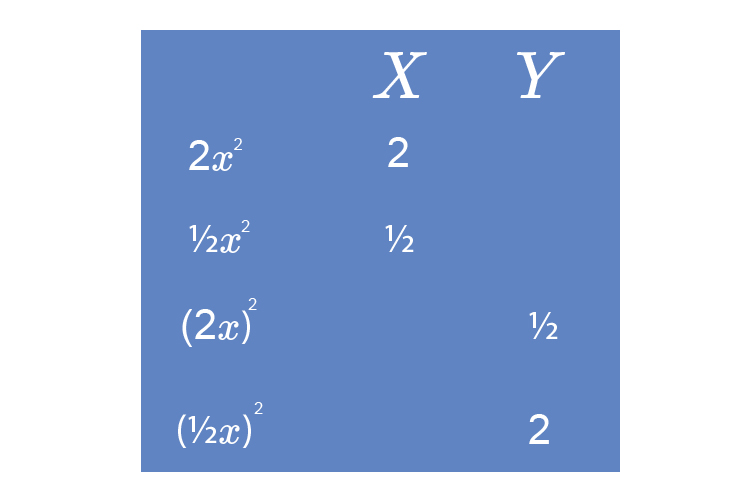# Enlarging or reducing parabolas

If you enlarge or reduce a parabola you make it steeper or flatter and there are four elements you need to remember.

y=2x^2          - Doubles the distance from the x  axis.

y=1/2x^2        - Halves the distance from the x  axis.

y=(2x)^2       - Halves the distance from the y  axis.

y=(1/2x)^2  - Doubles the distance from the y  axis.

We can remember this by using the following chart:Note also that

y=3x^2          - Triples the distance from the x  axis.

y=1/3x^2        - Thirds the distance from the x  axis.

y=(3x)^2       - Thirds the distance from the y  axis.

y=(1/3x)^2  - Triples the distance from the y  axis.

## y=x^2

y=x^2 should be plotted as:

 x=4 y=4^2 =16 x=3 y=3^2 =9 x=2 y=2^2 =4 x=1 y=1^2 =1 x=0 y=0^2 =0 x=-1 y=(-1)^2 =1 x=-2 y=(-2)^2 =4 x=-3 y=(-3)^2 =9 x=-4 y=(-4)^2 =16